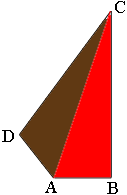SEARCH HOMEMath Central Quandaries & QueriesQuestion from Rahul, a student: find the area of the quadrilateral whose side measure9cm,40cm,28cm,15cm and in which the angle between the first 2 sides is a right angleRahul,

I drew a diagram (not to scale) of what you described. $|AB| = 9, |BC| = 40, |CD| = 28$ and $|DA| = 15$ centimeters.I joined $A$ to $C$ to partition the quadrilateral into 2 triangles. Triangle $ABC$ is a right triangle and its area is easy to calculate. Also since triangle;e $ABC$ is a right triangle you can use Pythagoras Theorem to find the length of the line segment $CA.$

You are left with triangle $CDA$ and you know the lengths of all three of its sides. To find its area look at the second paragraph in my response to Saba.

PennyMath Central is supported by the University of Regina and the Imperial Oil Foundation.